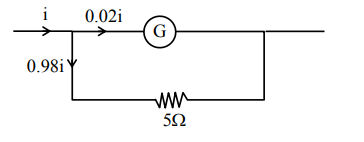# Consider a galvanometer shunted withQuestion:

Consider a galvanometer shunted with $5 \Omega$ resistance and $2 \%$ of current passes through it.

What is the resistance of the given galvanometer ?

1. $300 \Omega$

2.  $344 \Omega$

3. $245 \Omega$

4. $226 \Omega$

Correct Option: , 3

Solution:$0.02 \mathrm{i} \mathrm{Rg}=0.98 \mathrm{i} \times 5$

$R g=245 \Omega$

Option (3)